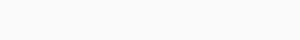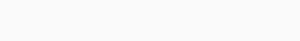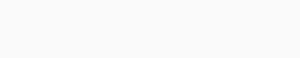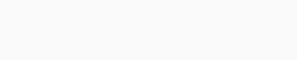# Understanding Capacitor Voltage Divider

A voltage divider is a passive linear circuit that exists in a capacitor. It produces an output voltage that is friction of its input voltage. Voltage divider circuits are constructed from reactive components just as they may be constructed from fixed value resistors.Capacitor voltage divider circuits are used in different electronics applications ranging from Colpitts Oscillator to capacitive touch-sensitive screens. This screen changes the output voltage when touched by a person’s finger. Also, it can be used as a cheap substitute for main transformers in dropping high voltages such as in mains connected circuits that use low voltage electronics or IC’s, etc.

Today we’ll be looking at the definition, formula, calculations, examples, and diagram of a capacitive voltage divider in a capacitor.

Contents

## Capacitive voltage divider

A capacitive voltage divider network cannot obstruct by changes in the supply frequency even though they use capacitors which are reactive elements. This work is just like resistive circuits. However, capacitors of the series chain, are affected equally by changes in supply frequency. One needs to under the capacitive reactance and how it affects capacitors at different frequencies to get the best of the article. In a previous post I enlighten on that, but let me make a quick recap.

Capacitors consist of two parallel conductive plates separated by an insulator. They have a positive (+) charge on one plate, and a negative (-) charge on the other. When connected to a DC supply, once the capacitor is fully charged, the insulator which serves as the dielectric material blocks the flow of current through it.

Just like a resistor, a capacitor opposes current flow, but stores energy on its plates when it charges and releases or gives back the energy into the connected circuit when it discharges. Resistors dissipate their unwanted energy in form of heat. Let take a look at how voltage distribution in series capacitors forming a capacitive voltage divider circuit.

Read more: Understanding the charge in a capacitor

Considering the two capacitors, C1 and C2 connected in series across an alternating supply of 10 volts. Since the two capacitors are in series, they carry the same charge Q. However, the voltage across them will be different and related to their capacitance values, as V = Q/C.Using the above diagram as an example, voltage divider circuits may be constructed from reactive components. The voltage across each capacitor can be calculated in different ways. One way is to find the capacitive reactance value of each capacitor, the total circuit impedance, the circuit current, use them to calculate the voltage drop. For instance:

## The first example of a capacitive voltage divider

Having the two capacitors of 10uF and 22UF just as in the series circuit above, calculate the RMS voltage drops across each capacitor when subjected to a sinusoidal voltage of 10 volts RMS at 80Hz.

Read more: Understanding capacitance in AC circuits

### Solution:

#### Capacitive Reactance of 10uF capacitor#### Capacitive Reactance of 22uF capacitorTotal capacitive reactance of series circuit – Note that reactances in series are added together just like resistors in series.or#### Circuit currentThen the voltage drop across each capacitor in series capacitive voltage divider will be:

You should know when the capacitor values are different, the smaller value capacitor will charge itself to a higher voltage than the large value capacitor. In the above example, this was 6.9 and 3.1 volts respectively. Because Kirchhoff’s voltage law applies to this and the series-connected circuit, the total sum of the individual voltage drops will be equal in value to the supply voltage, Vs and 6.9 + 3.1 are equal to 10 volts.

You should also know the ratios of the voltage drops across the two capacitors connected in a series capacitive voltage divider circuit will always remain the same regardless of the supply frequency. So, the voltage drops of 6.9 volts and 3.1 volts in the example will remain the same even if the supply frequency is increased from 80Hz to 8000Hz as shown.

### The second example of a capacitive voltage dividerUsing the same two capacitors, calculate the capacitive voltage drop at 8,000Hz (8kHz).

As the voltage ratio across the two capacitors stays the same and the supply frequency increases, the combined capacitive reactance decreases. Also, the total circuit impedance decreases, therefore, the reduction in impedance causes more current to flow. For instance, at 80Hz we calculated the circuit current above to be about 34.4mA, but at 8kHz, the supply current increased to 3.4A, 100 times more. Thus, the current flowing through a capacitive voltage divider is proportional to frequency or I ∝ ƒ.

You should know that a capacitor divider is a network of series-connected capacitors having AC voltage drop across it. The capacitor voltage dividers use the capacitive reactance value of a capacitor to determine the actual voltage drop. They can only be used on frequency-driven supplies and they do not work as DC voltage dividers. This is mainly because the capacitors block DC and allows no current to flow.

#### Watch the video below to learn more on how to calculate capacitor voltage dividers:

Just as you’ve learned, the reactance of both capacitors changes with frequency (at the same rate), the voltage division across a capacitive voltage divider circuit will always remain the same sustaining a steady voltage divider. That is all for this article, where the definition, formula, calculations, examples, and diagram of capacitor voltage divider are being discussed.  I hope you gain a lot from the reading, if so, kindly share with other students. Thanks for reading, see you next time!